• 创建三维饼图
2021-07-20 00:35:17

【摘要】环球网校分享的2017年职称计算机考试Excel考点：创建饼图图表希望对大家备考2017年职称计算机考试有帮助，更多资料敬请关注环球职称计算机考试频道，网校会及时更新考试资讯……　　相关推荐：2017年

【摘要】环球网校分享的“2017年职称计算机考试Excel考点：创建饼图图表”希望对大家备考2017年职称计算机考试有帮助，更多资料敬请关注环球职称计算机考试频道，网校会及时更新考试资讯……

创建饼图图表工作表并设置图表工作表的格式(环球网校分享的2017年职称计算机考试Excel考点：创建饼图图表)

打开工作簿“cunkuan.xls”，将“家庭存款账单”工作表中的数据按“存款银行”分类汇总“本息”字段，汇总方式为“求和”，然后建立一个三维饼图图表工作表。建立要求：

①系列：各项汇总的本息和。

②数据标志：各个存款银行。

③图表标题：各银行本息比例;无图例;同时显示百分比及数据标志。

④将三维饼图图表工作表命名为“各银行本息比例”。

操作方法如下：

①打开工作簿“cunkuan.xls”，将“家庭存款账单”工作表中的数据按“存款银行”分类汇总“本息”字段，汇总方式为“求和”;单击“2级显示”按钮，将分类汇总结果折叠。

②单击“常用”工具栏中的“图表向导”按钮，打开“图表向导-4步骤之1-图表类型”对话框，在“图表类型”列表框中选择“饼图”类型，在“子图表类型”列表框中选择“三维饼图”子图类型。

③单击“下一步”按钮，打开“图表向导-4步骤之2-图表数据源”对话框，单击“系列”选项卡，在此对话框中设置以下参数：(环球网校分享的2017年职称计算机考试Excel考点：创建饼图图表)

a)单击“名称”文本框右侧的折叠按钮，再单击字段名称“本息”;单击“值”文本框右侧的折叠按钮，再选中“本息”字段中的汇总数据区域F6:F18。 b)单击“分类标志”框右侧的折叠按钮，选中“存款银行”所在列的数据区域H6:H18(注意：不包括字段名)。

编辑推荐：

环球网校友情提示：“2017年职称计算机考试Excel考点：创建饼图图表”，如果您在此过程中遇到任何疑问，请登录环球网校职称计算机频道及职称计算机论坛，我们随时与广大考生朋友们一起交流!

更多相关内容
• 本代码主要利用MATLAB工具实现MATLAB——创建二维饼图和三维饼图，简单明了，易于理解
• 创建销售份额三维饼图.xlsx源码EXCEL VBA宏编程xlsx实例代码下载创建销售份额三维饼图.xlsx源码EXCEL VBA宏编程xlsx实例代码下载创建销售份额三维饼图.xlsx源码EXCEL VBA宏编程xlsx实例代码下载创建销售份额三维饼图...
• pct(x/sum(x)*100) lbls2(lbls,"",pct,"%",sep="")#表示标签的形式 pie(x,labels=lbls2,col=rainbow(length(lbls2)),main=“Pie Chart with Percentages”) #三维饼图的设置 使用plotrix包中的pie3D()函数来创建 ...
	R	语言关于棘状图，以及饼图，三维饼图的画法


使用vcd包中的spine()函数进行绘制
library(vcd)
attach(Arthritis)
counts<-table(Treatment,Improved)
spine(counts,main=“Spinogram Example”)
detach(Arthritis)饼图的画法以及三维饼图的画法
##饼图的画法利用函数pie(x,label),其中x表示的是label 所占的比重

par(mfrow=c(2,2))
x<-c(10,12,4,16,8)
lbls<-c(“US”,“UK”,“Australia”,“Germany”,“France”)
pie(x,labels=lbls,main=“Sample Pie Chart”)

##为饼图添加比例数据
pct<-round(x/sum(x)*100)
lbls2<-paste(lbls,"",pct,"%",sep="")#表示标签的形式
pie(x,labels=lbls2,col=rainbow(length(lbls2)),main=“Pie Chart with Percentages”)
#三维饼图的设置
使用plotrix包中的pie3D()函数来创建
library(plotrix)
pie3D(x,labels=lbls,explode=0.1,main=“3D Pie Chart”)#explode表示不同区域分开的距离
mytable<-table(state.region)
lbls3<-paste(names(mytable),"\n",mytable,sep="")#将字符转换为向量
pie(mytable,labels=lbls3,main=“Pie Chart from Table\n
(with sample sizes)”)展开全文r语言
• 最近有多位读者反应，3D 饼图在 VUE 环境...这两天有空，为了看看到底是什么原因，我跑去查了查 VUE 的手册和教程，尝试在 @vue/cli 创建的 webpack 中，把我的 3D 饼图跑通。最终效果如下：最终效果下文主要分为...

最近有多位读者反应，3D 饼图在 VUE 环境里跑不通。可我其实是一个先学 ECharts 后学 Javascript 的前端外行，再加上读者往往也没有告诉我具体的报错信息是什么，所以一时也没能帮读者找出问题原因。

这两天有空，为了看看到底是什么原因，我跑去查了查 VUE 的手册和教程，尝试在 @vue/cli 创建的 webpack 中，把我的 3D 饼图跑通。最终效果如下：最终效果

下文主要分为三大块：环境准备(node.js、vue、webpack、@vue/cli 安装等)

创建 VUE 项目，安装依赖

App.vue 文件编写

环境准备(前端们见笑了，可以略过)安装 Node.js

建议跟我一样的前端外行，直接使用包管理器安装，具体搜索「Installing Node.js via package manager」吧，我之前用的好像是「yum」，安装后使用命令：

node -vNode.js 安装结果

查看安装结果。

安装 @vue/cli

Node.js 安装好之后，使用命令：

npm install -g @vue/cli

安装「Vue-CLI」，完成后使用命令「vue -V」查看安装结果。@vue/cli 安装结果

创建项目

「Vue-CLI」安装后，就可以使用「vue create xxx」命令创建项目(我选择的「Vue 2」)，xxx 是项目名称，也是自动创建的项目目录名。选择 Vue 2项目创建完成

项目创建完成，按提示跑一下先看看「cd xxx&&npm run serve」浏览器访问效果

安装 ECharts 相关依赖

在项目目录中执行命令

npm install echarts@4.9.0 echarts-gl vue-echarts –-save

安装所需的 ECharts 依赖。Vue-ECharts

Apache ECharts (incubating) component for Vue.js.App.vue 文件编写

我就是参考那个文件，改写我的 3D 饼图的。有兴趣的同学可以自行尝试一下，也可以在文章尾部复制，保存为「App.vue」,替换掉 src 目录下的 App.vue。

改写/替换好之后，再次运行项目浏览器访问效果App.vue 内容简要解释

从文档中查到，扩展名为 .vue 的文件被称为 single-file components (单文件组件)，而这个 App.vue 又有点像 Express 的 app.js，似乎是整个项目的入口文件。作为新手，为了省事，我直接把内容写到了 App.vue 中…通过 引入 vue-echarts 组件(注册是在后面的标签内)，并绑定数据、绑定事件:options="pie3D"：也就是 v-bind:options="pie3D"，这个 options 就是 ECharts 实例的数据，修改这个参数会触发 ECharts 实例的 setOption 方法。

注意：如果直接修改 options 绑定的数据而对象引用保持不变，setOption 方法调用时将带有参数 notMerge: false。否则，如果为 options绑定一个新的对象，setOption 方法调用时则将带有参数 notMerge: true。

ref="pie3D"：ref 被用来给元素或子组件注册引用信息。

:manual-update=true：当将 manual-update prop 指定为 true 且不传入 options prop 时，数据将不会被监听。然后，你需要用 ref 获取组件实例以后手动调用 mergeOptions 方法来更新图表。

@click="onClick"：也就是 v-on:click="onClick"，为 click 事件绑定 onCLick 方法，而方法也是在后面的

后略(同上)

​在标签中编写 Javascript 代码，先 import 所需的依赖，再定义一些函数(这几个函数基本都没有改动)

其中 onClick、onMouseover、onGlobalout 这三个函数，是根据之前 Gallery 中例子里的，三个 mychart.on('xxx',handler) 改写的。主要改动是通过 this.pie3D 获取、更新饼图当前的 option 数据，通过 mergeOptions 方法刷新图表。之前的 3D 饼图文章​zhuanlan.zhihu.com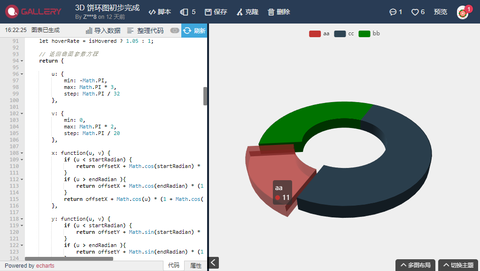另外，有些读者在 Gallery 看到的例子可能是基于我这个改写的，增加了单独设置高度的功能：回复评论：能单独调每一块内容的高度吗..有高有低的那种

你们都太有想法了~把z:function(u,v){}里面的返回值，都乘以一个系数就可以，然后这个系数作为getParametricEquation函数的入参传进来。(当然后面那些高亮、放大效果也得跟着改一下)

附件：pie3D 的 App.vue 完整代码

:options="pie3D"

ref="pie3D"

:manual-update=true

@click="onClick"

@mouseover="onMouseover"

@globalout="onGlobalout"

/>

/*** 默认尺寸为 600px×400px，如果想让图表响应尺寸变化，可以像下面这样* 把尺寸设为百分比值(同时请记得为容器设置尺寸)。*/

/**.echarts {width: 100%;height: 100%;}*/

import ECharts from 'vue-echarts'

//import 'echarts/lib/chart/line'//import 'echarts/lib/component/polar'import 'echarts-gl'

// 生成扇形的曲面参数方程，用于 series-surface.parametricEquationfunction getParametricEquation(startRatio, endRatio, isSelected, isHovered, k) {

// 计算 let midRatio = (startRatio + endRatio) / 2;

let startRadian = startRatio * Math.PI * 2;

let endRadian = endRatio * Math.PI * 2;

let midRadian = midRatio * Math.PI * 2;

// 如果只有一个扇形，则不实现选中效果。 if (startRatio === 0 && endRatio === 1) {

isSelected = false;

}

// 通过扇形内径/外径的值，换算出辅助参数 k(默认值 1/3) k = typeof k !== 'undefined' ? k : 1 / 3 ;

// 计算选中效果分别在 x 轴、y 轴方向上的位移(未选中，则位移均为 0) let offsetX = isSelected ? Math.cos(midRadian) * 0.1 : 0;

let offsetY = isSelected ? Math.sin(midRadian) * 0.1 : 0;

// 计算高亮效果的放大比例(未高亮，则比例为 1) let hoverRate = isHovered ? 1.05 : 1;

// 返回曲面参数方程 return {

u: {

min: -Math.PI,

max: Math.PI * 3,

step: Math.PI / 32

},

v: {

min: 0,

max: Math.PI * 2,

step: Math.PI / 20

},

x: function(u, v) {

if (u < startRadian) {

return offsetX + Math.cos(startRadian) * (1 + Math.cos(v) * k) * hoverRate;

}

if (u > endRadian ){

return offsetX + Math.cos(endRadian) * (1 + Math.cos(v) * k) * hoverRate;

}

return offsetX + Math.cos(u) * (1 + Math.cos(v) * k) * hoverRate;

},

y: function(u, v) {

if (u < startRadian) {

return offsetY + Math.sin(startRadian) * (1 + Math.cos(v) * k) * hoverRate;

}

if (u > endRadian ){

return offsetY + Math.sin(endRadian) * (1 + Math.cos(v) * k) * hoverRate;

}

return offsetY + Math.sin(u) * (1 + Math.cos(v) * k) * hoverRate;

},

z: function(u, v) {

if (u < - Math.PI * 0.5 ) {

return Math.sin(u);

}

if (u > Math.PI * 2.5 ){

return Math.sin(u);

}

return Math.sin(v) > 0 ? 1 : -1;

}

};

}

// 生成模拟 3D 饼图的配置项function getPie3D(pieData, internalDiameterRatio) {

let series = [];

let sumValue = 0;

let startValue = 0;

let endValue = 0;

let legendData = [];

let k = typeof internalDiameterRatio !== 'undefined' ? (1 - internalDiameterRatio) / (1 + internalDiameterRatio): 1 / 3;

// 为每一个饼图数据，生成一个 series-surface 配置 for (let i = 0; i < pieData.length; i++) {

sumValue += pieData[i].value;

let seriesItem = {

name: typeof pieData[i].name === 'undefined' ? series${i} : pieData[i].name, type: 'surface', parametric: true, wireframe: { show: false }, pieData: pieData[i], pieStatus: { selected: false, hovered: false, k: k } }; if (typeof pieData[i].itemStyle != 'undefined') { let itemStyle = {}; typeof pieData[i].itemStyle.color != 'undefined' ? itemStyle.color = pieData[i].itemStyle.color : null; typeof pieData[i].itemStyle.opacity != 'undefined' ? itemStyle.opacity = pieData[i].itemStyle.opacity : null; seriesItem.itemStyle = itemStyle; } series.push(seriesItem); } // 使用上一次遍历时，计算出的数据和 sumValue，调用 getParametricEquation 函数， // 向每个 series-surface 传入不同的参数方程 series-surface.parametricEquation，也就是实现每一个扇形。 for (let i = 0; i < series.length; i++) { endValue = startValue + series[i].pieData.value; series[i].pieData.startRatio = startValue / sumValue; series[i].pieData.endRatio = endValue / sumValue; series[i].parametricEquation = getParametricEquation(series[i].pieData.startRatio, series[i].pieData.endRatio, false, false, k); startValue = endValue; legendData.push(series[i].name); } // 补充一个透明的圆环，用于支撑高亮功能的近似实现。 series.push({ name: 'mouseoutSeries', type: 'surface', parametric: true, wireframe: { show: false }, itemStyle: { opacity: 0 }, parametricEquation: { u: { min: 0, max: Math.PI * 2, step: Math.PI / 20 }, v: { min: 0, max: Math.PI, step: Math.PI / 20 }, x: function(u, v) { return Math.sin(v) * Math.sin(u) + Math.sin(u); }, y: function(u, v) { return Math.sin(v) * Math.cos(u) + Math.cos(u); }, z: function(u, v) { return Math.cos(v) > 0 ? 0.1 : -0.1; } } }); // 准备待返回的配置项，把准备好的 legendData、series 传入。 let option = { //animation: false, legend: { data: legendData }, tooltip: { formatter: params => { if (params.seriesName !== 'mouseoutSeries') { return ${params.seriesName}
${option.series[params.seriesIndex].pieData.value}; } } }, xAxis3D: { min: -1, max: 1 }, yAxis3D: { min: -1, max: 1 }, zAxis3D: { min: -1, max: 1 }, grid3D: { show: false, boxHeight: 10, //top: '30%', bottom: '50%' }, series: series }; return option; } // 传入数据生成 optionlet option = getPie3D([{ name: 'aa', value: 11, itemStyle: { opacity: 0.5 } }, { name: 'cc', value: 33 }, { name: 'bb', value: 22, itemStyle: { color: 'green' } }]); export default { components: { 'v-chart': ECharts }, data () { return { pie3D: option, selectedIndex: '', hoveredIndex: '' } }, methods: { onClick(params) { // 点击透明圆环时不做操作 if (params.seriesName === 'mouseoutSeries') { return } //【VUE 新增】深拷贝现有 option let option = JSON.parse(JSON.stringify(this.pie3D)) let isSelected = !option.series[params.seriesIndex].pieStatus.selected; let isHovered = option.series[params.seriesIndex].pieStatus.hovered; let k = option.series[params.seriesIndex].pieStatus.k; let startRatio = option.series[params.seriesIndex].pieData.startRatio; let endRatio = option.series[params.seriesIndex].pieData.endRatio; // 如果之前选中过其他扇形，将其取消选中(对 option 更新) if (this.selectedIndex !== '' && this.selectedIndex !== params.seriesIndex) { option.series[this.selectedIndex].parametricEquation = getParametricEquation(option.series[this.selectedIndex].pieData.startRatio, option.series[this.selectedIndex].pieData.endRatio, false, false, k); option.series[this.selectedIndex].pieStatus.selected = false; } // 对当前点击的扇形，执行选中/取消选中操作(对 option 更新) option.series[params.seriesIndex].parametricEquation = getParametricEquation(startRatio, endRatio, isSelected, isHovered, k); option.series[params.seriesIndex].pieStatus.selected = isSelected; // 如果本次是选中操作，记录上次选中的扇形对应的系列号 seriesIndex isSelected ? this.selectedIndex = params.seriesIndex : null; // 使用更新后的 option，渲染图表 //myChart.setOption(option); //【VUE 新增】修改 data.pie3D 并更新图表(用 ref 获取组件实例以后手动调用 mergeOptions 方法来更新图表，也可以不设置 manual-update 为 true，直接修改 this.pie3D 触发更新) this.pie3D = JSON.parse(JSON.stringify(option)) this.$refs.pie3D.mergeOptions(option)

},

onMouseover(params) {

//【VUE 新增】深拷贝现有 option let option = JSON.parse(JSON.stringify(this.pie3D))

// 准备重新渲染扇形所需的参数 let isSelected;

let isHovered;

let startRatio;

let endRatio;

let k;

// 如果触发 mouseover 的扇形当前已高亮，则不做操作 if (this.hoveredIndex === params.seriesIndex) {

return;

// 否则进行高亮及必要的取消高亮操作 } else {

// 如果当前有高亮的扇形，取消其高亮状态(对 option 更新) if (this.hoveredIndex !== '') {

// 从 option.series 中读取重新渲染扇形所需的参数，将是否高亮设置为 false。 isSelected = option.series[this.hoveredIndex].pieStatus.selected;

isHovered = false;

startRatio = option.series[this.hoveredIndex].pieData.startRatio;

endRatio = option.series[this.hoveredIndex].pieData.endRatio;

k = option.series[this.hoveredIndex].pieStatus.k;

// 对当前点击的扇形，执行取消高亮操作(对 option 更新) option.series[this.hoveredIndex].parametricEquation = getParametricEquation(startRatio, endRatio, isSelected, isHovered, k);

option.series[this.hoveredIndex].pieStatus.hovered = isHovered;

// 将此前记录的上次选中的扇形对应的系列号 seriesIndex 清空 this.hoveredIndex = '';

}

// 如果触发 mouseover 的扇形不是透明圆环，将其高亮(对 option 更新) if (params.seriesName !== 'mouseoutSeries') {

// 从 option.series 中读取重新渲染扇形所需的参数，将是否高亮设置为 true。 isSelected = option.series[params.seriesIndex].pieStatus.selected;

isHovered = true;

startRatio = option.series[params.seriesIndex].pieData.startRatio;

endRatio = option.series[params.seriesIndex].pieData.endRatio;

k = option.series[params.seriesIndex].pieStatus.k;

// 对当前点击的扇形，执行高亮操作(对 option 更新) option.series[params.seriesIndex].parametricEquation = getParametricEquation(startRatio, endRatio, isSelected, isHovered, k);

option.series[params.seriesIndex].pieStatus.hovered = isHovered;

// 记录上次高亮的扇形对应的系列号 seriesIndex this.hoveredIndex = params.seriesIndex;

}

// 使用更新后的 option，渲染图表 // myChart.setOption(option);

//【VUE 新增】修改 data.pie3D 并更新图表(用 ref 获取组件实例以后手动调用 mergeOptions 方法来更新图表，也可以不设置 manual-update 为 true，直接修改 this.pie3D 触发更新) this.pie3D = JSON.parse(JSON.stringify(option))

this.$refs.pie3D.mergeOptions(option) } }, onGlobalout() { //【VUE 新增】深拷贝现有 option let option = JSON.parse(JSON.stringify(this.pie3D)) if (this.hoveredIndex !== '') { // 从 option.series 中读取重新渲染扇形所需的参数，将是否高亮设置为 true。 let isSelected = option.series[this.hoveredIndex].pieStatus.selected; let isHovered = false; let k = option.series[this.hoveredIndex].pieStatus.k; let startRatio = option.series[this.hoveredIndex].pieData.startRatio; let endRatio = option.series[this.hoveredIndex].pieData.endRatio; // 对当前点击的扇形，执行取消高亮操作(对 option 更新) option.series[this.hoveredIndex].parametricEquation = getParametricEquation(startRatio, endRatio, isSelected, isHovered, k); option.series[this.hoveredIndex].pieStatus.hovered = isHovered; // 将此前记录的上次选中的扇形对应的系列号 seriesIndex 清空 this.hoveredIndex = ''; } // 使用更新后的 option，渲染图表 //myChart.setOption(option); //【VUE 新增】修改 data.pie3D 并更新图表(用 ref 获取组件实例以后手动调用 mergeOptions 方法来更新图表，也可以不设置 manual-update 为 true，直接修改 this.pie3D 触发更新) this.pie3D = JSON.parse(JSON.stringify(option)) this.$refs.pie3D.mergeOptions(option)

}

}

}

参考

展开全文3d饼图 vue
• 然后使用下面两种方式之一声明要创建三维子图 ax = fig.gca(projection='3d') ax = plt.subplot(111,projection='3d') 接下来就可以使用ax的plot（）方法绘制三维曲线，plt_surface()方法绘制三维曲面，...

## 雷达图

雷达图又称戴布拉图、蜘蛛网图，可以很好刻画出某些指标的横向或纵向的对比关系

雷达图通常用于多想指标的全面分析。 比如：HR想要比较两个应聘者的综合素质，用雷达图分别画出来，就可以进行直观的比较

python中matplotlib模块绘制雷达图需要用到极坐标系。

### 雷达图之极坐标系

在平面内去一个定点O,叫极点，引一条射线Ox，叫做极轴，在选定一个长度单位和角度的正方向（通常去逆时针方向）。对于平面内任何一点M，用p表示线段OM的长度（有时也用r），の表示从Ox到OM的角度，p叫做点M的极径，の叫做点M的极角，有序数对（p，の）就叫做点M的极坐标，这样建立的坐标系叫做极坐标系。

通常情况下，M的极径坐标单位为1，极角坐标单位为1°。

### 雷达图的polar函数

polar(theta,r,**kwargs)


主要参数：

theta：极角の

r：极径

1.普通小图

import matplotlib.pyplot as plt
import numpy as np

plt.polar(0.25*np.pi,20,'ro',lw=2) # r表示红色，o是圆点 lw宽度为2
plt.ylim(0,50)  # 设置极轴的上下限
plt.show()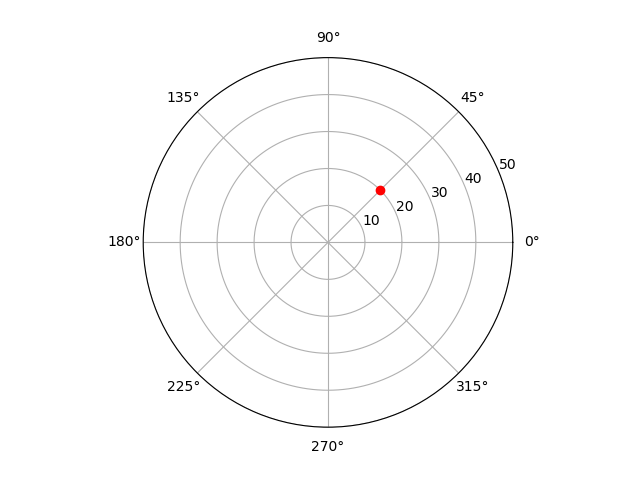2.如果绘制多个极角和极轴时：

theta：定义了一个ndarry数组存储多个数据。

r：定义一个数组存放极轴的长度，也叫极径。

import matplotlib.pyplot as plt
import numpy as np

theta = np.array([0.25,0.5,0.75,1,1.25,1.5,1.75,2])
r = [75,25,40,64,58,14,80,29]
plt.polar(theta*np.pi,r,'ro',lw=2)
plt.ylim(0,100)
plt.show()

# 在图中绘制出多个点：(0.25*π,75),(0.5*π,25).....的点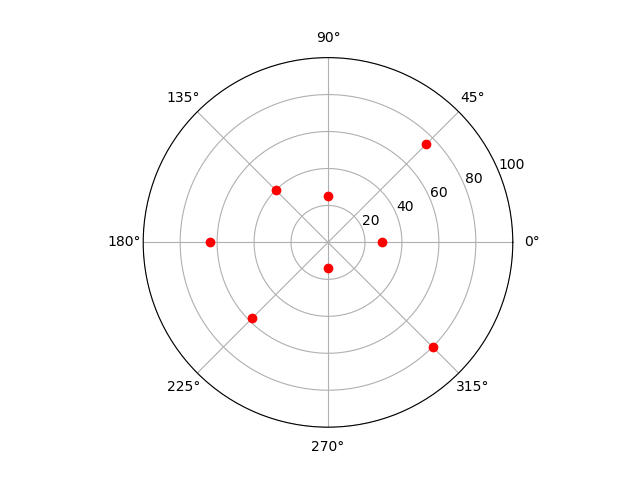3.用线把每个点连起来

只需要在样式里面，把‘ro’改成‘ro-’

import matplotlib.pyplot as plt
import numpy as np

theta = np.array([0.25,0.5,0.75,1,1.25,1.5,1.75,2])
r = [75,25,40,64,58,14,80,29]
plt.polar(theta*np.pi,r,'ro-',lw=2)
plt.ylim(0,100)
plt.show()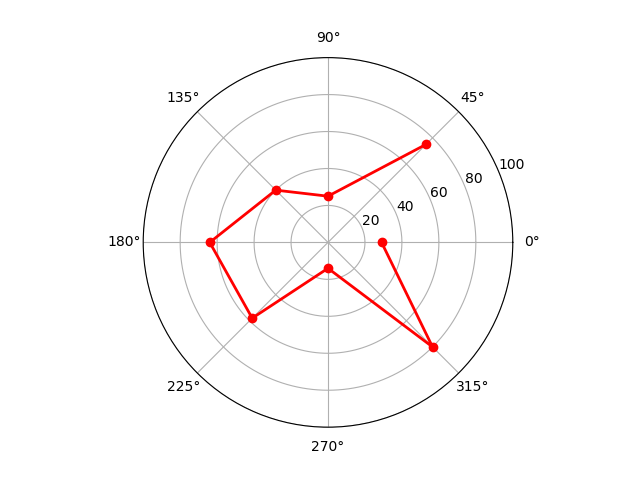上面看到我们这个并不是闭合的曲线

4.构造闭合的曲线

只需要多构造一个极坐标点，和第一个重叠就好

import matplotlib.pyplot as plt
import numpy as np

theta = np.array([0.25,0.5,0.75,1,1.25,1.5,1.75,2,0.25])
r = [75,25,40,64,58,14,80,29,75]
plt.polar(theta*np.pi,r,'ro-',lw=2)
plt.ylim(0,100)
plt.show()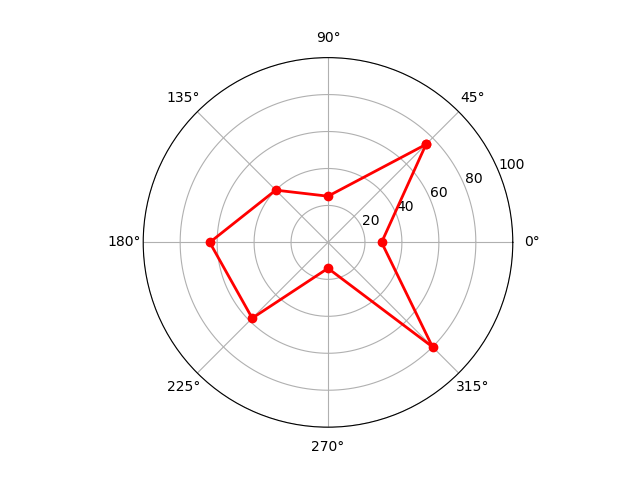5.填充颜色

fill（）函数来填充雷达图

import matplotlib.pyplot as plt
import numpy as np

theta = np.array([0.25,0.5,0.75,1,1.25,1.5,1.75,2,0.25])
r = [75,25,40,64,58,14,80,29,75]
plt.polar(theta*np.pi,r,'ro-',lw=2)
plt.fill(theta*np.pi,r,facecolor='r',alpha=0.25)
plt.ylim(0,100)
plt.show()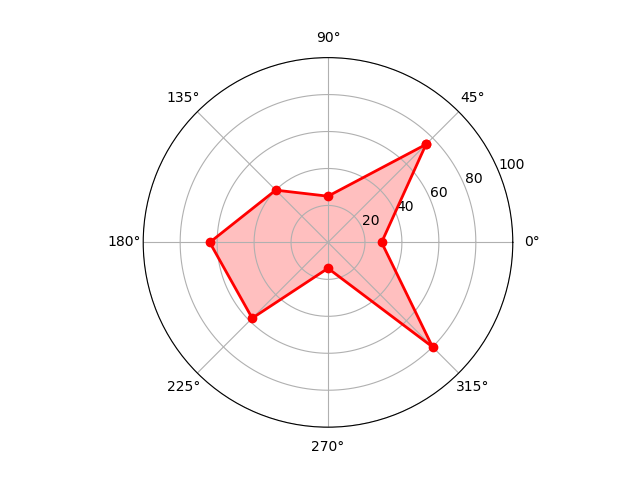## 雷达图实战：

利用linspace将圆等分，

linspace(start, stop, num=50, endpoint=True, retstep=False, dtype=None, axis=0)
初始数据，结束数据，数据个数，endpoin：是否包含终点

import matplotlib.pyplot as plt
import numpy as np

course = ['C++','Python','高等数学','大学英语','软件工程','组成原理','操作系统','网络工程']
scores = [82,95,95,89,85,94,86,99]

dataLength = len(scores)

# 把园等分为dataLength份
angles = np.linspace(0,2*np.pi,dataLength,endpoint=False)
scores.append(scores)
angles = np.append(angles,angles)
plt.polar(angles,scores,'rv--',lw=2)
plt.thetagrids(angles*180/np.pi,course,fontproperties = 'simhei')
plt.fill(angles,scores,facecolor='r',alpha = 0.4)
plt.show()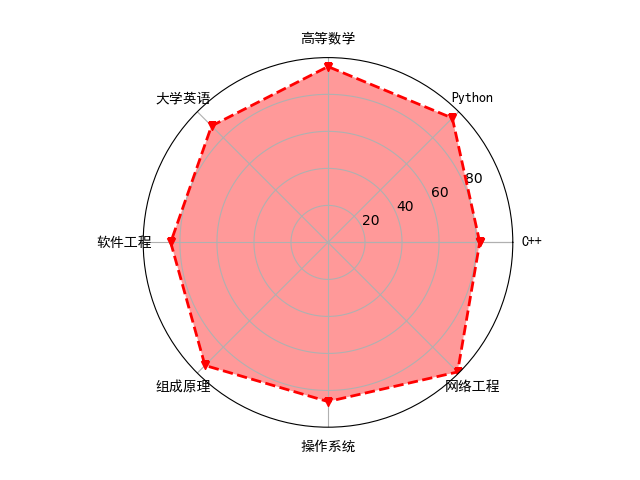## 三维图

matplotlib支持一些基础的三维图的绘制，比如说曲面散点图和柱状图，需要使用mpl_toolkits模块

如果要绘制三维图形，首先需要使用下面的语句导入相应的对象：

from mpl_toolkits.mplot3d import Axes3D


然后使用下面两种方式之一声明要创建的三维子图

ax = fig.gca(projection='3d')

ax = plt.subplot(111,projection='3d')


接下来就可以使用ax的plot（）方法绘制三维曲线，plt_surface()方法绘制三维曲面，scatter()方法绘制三维散点图或bar3d（）方法绘制三维柱状图了。

### 三维曲面的绘制方法：p3d.Axes3D.plot_surface()

在绘制三维图形时，至少小指定x，y，z三个坐标的数据，然后再根据不同的图形类型指定额外的参数设置图形的属性。

plot_surface(X,Y,Z,*args,**kwargs)


常用参数：

rstride和csride分别控制xy两个方向的步长，这决定了曲面上每个面的大小

color：指定面片的颜色

cmap：指定面片的颜色映射表

### 三维散点图绘制方法：p3d.Axes3D.scatter()

p3d.Axes3D.scatter(xs, ys, zs=0, zdir='z', s=20, c=None, depthshade=True, *args, **kwargs)


常用参数：

xs,ys,zs分别用来指定散点符号的xyz轴，如果同时为标量则指定一个散点符号的坐标，如果同时为等长数组则指定一系列散点符号的坐标

s用来指定散点符号的大小，可以是标量或与xs等长的数组

### 三维柱状图的绘制方法：p3d.Axes3D.bar3d()

bar3d(self, x, y, z, dx, dy, dz, color=None, zsort='average', shade=True, *args, **kwargs)


常用参数：

x，t，z：分别用来指定每个柱地面的坐标，如果这个三个参数都是标量则指定一个柱的底面坐标，如果是三个等长的数组则指定多个柱的底面坐标

dx，dy，dz：分别用来指定柱在三个坐标轴上的跨度，即x方向的宽度，y方向的厚度和z方向的高度。

color：指定柱的表面颜色

### 三维曲面图实战

根据下面的测试数据展示图片

theta = np.linspace(-4np.pi,4np.pi,100)
z = np.linspace(-4,4,100)0.3
r = z**4+1
x = r
np.sin(theta)
y = r*np.cos(theta)

import matplotlib as mpl
import numpy as np
from mpl_toolkits.mplot3d import Axes3D
import matplotlib.pyplot as plt
import matplotlib.font_manager as fm

#绘制三维图形
fig = plt.figure() # 初始化
ax = fig.gca(projection = '3d') # 设置3d属性

#生成测试数据
theta = np.linspace(-4*np.pi,4*np.pi,100)
z = np.linspace(-4,4,100)*0.3
r = z**4+1
x = r*np.sin(theta)
y = r*np.cos(theta)

#绘制三维曲线 设置标签
ax.plot(x,y,z,'bv-',label='参数曲线')

# 设置图例字体 字号 显示图例
font = fm.FontProperties(fname=r'C:\Windows\Fonts\simhei.ttf') # 可以不设置
mpl.rcParams['legend.fontsize']=10 # 设置字号
ax.legend(prop=font)
plt.show()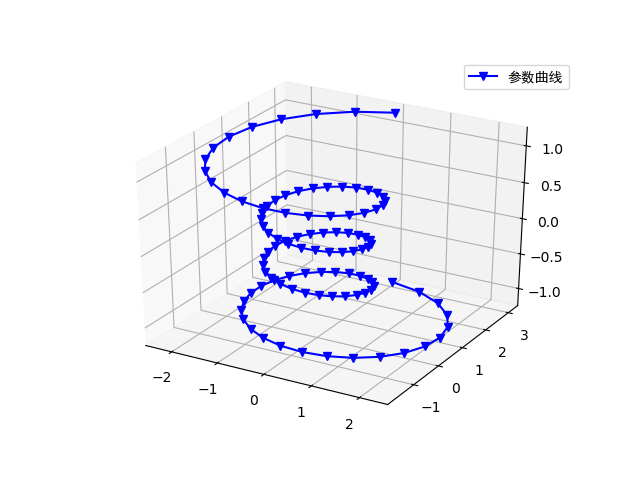### 三维柱状图实战

import numpy as np
from mpl_toolkits.mplot3d import Axes3D
import matplotlib.pyplot as plt

x = np.random.randint(0,40,10)
y = np.random.randint(0,40,10)
z = 80*abs(np.sin(x+y))

ax = plt.subplot(projection = '3d')

for xx,yy,zz in zip(x,y,z):
color = np.random.random(3)
ax.bar3d(xx,yy,0,dx=1,dy=1,dz=zz,color = color)
ax.set_xlabel('X')
ax.set_ylabel('Y')
ax.set_zlabel('Z')

plt.show()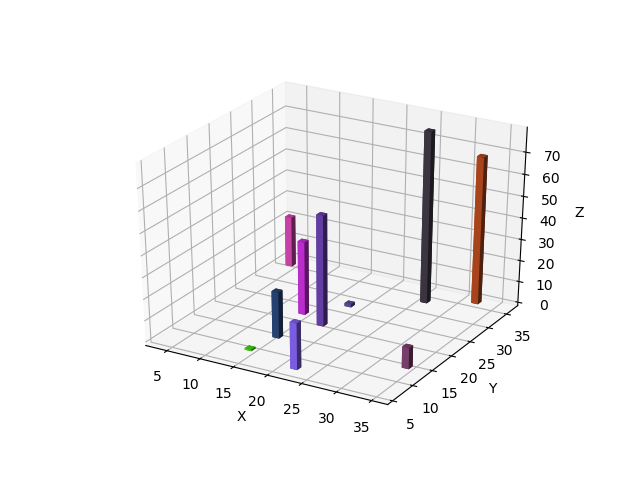如果想获取更多有关python的信息，和想玩python制作的小程序，可以关注微信公众号（dreamspy）。我们一起用python改变世界，一起用python创造梦想。展开全文python
• } privatePoint2D calculateArcMiddle() { // 创建一个新的弧，其扩展角度为当前弧的一半 returnnewArc2D.Double(arc.getX(),arc.getY(),arc.getWidth(),arc.getHeight(), arc.getAngleStart(),arc.getAngleExtent...java 饼图
• ECharts 提供了常规的折线图、柱状图、散点图、饼图、K线图，用于统计的盒形图，用于地理数据可视化的地图、热力图、线图，用于关系数据可视化的关系图、treemap、旭日图，多维数据可视化的平行坐标，还有用于 BI ...
• 项目中用过，3D阶梯图 3D饼状图 three.js 实现，二数据展示 修改数据中的heightValue都等于一样的值，就变成了3D饼图了。 如有其他问题可以评论，我看到了会及时解决。谢谢。
• 第一关：绘制三维图 任务描述 本关任务：使用matplotlib绘制三维图。 相关知识 matplotlib原本只能画二维图，随着版本更新matplotlib实现了一些建立在二维图上的三维图。 话不多说，直接代码 import ...r语言 python
• 形象的三维饼图。 向窗体上添加一个tvtchart组件和一个 ttimer 组件,其中tvtchart组件用于显示饼图,而ttimer组件用于不停地改变饼图的数据,获得动态的效果。设计完成的主界面如图1所示。 图1 主界面 ...timer random math
• 之前使用class组件的方式创建三维应用，今天试着使用react hooks创建function 组件。 function组件与class组件最大的不同是function组件能够捕获渲染的值，而class组件因为react中this是可变的， 所以总是能获取...
• 在我们使用excel表格统计数据时，可以制作各种各样的图形来统计，比如说饼图、折线图、瀑布图等等，近日很多用户比较常用三维簇状柱形图，看到它的名字就知道不简单，那么excel怎么制作三维簇状柱形图呢?其实很简单...
• 课时20 21、添加三维文字 07:20 课时21 22、创建书柜 07:52 课时22 23、创建茶机 07:38 课时23 24、镜头和视图工具 06:21 课时24 25、等高线创建、网格创建、曲面起伏工具 06:20 课时25 26、曲成投射工具 03:22 课时...
• matlab画饼图函数：pie()二维饼图，pie3()三维饼图，下面以pie3为例具体介绍一下函数用法（pie函数用法相同）。 pie3(x) 1、当X中元素的和大于1时，则pie3通过X/sum(X)对值进行归一化，以确定饼中的每个切片的面积。...matlab
• matlab实验三二维图形和三维图形的创建_计算机软件及应用_IT/计算机_专业资料。MATLAB二维图形的绘制,图形的标注,三维曲线和曲面图形的绘制。 实验三:二维图形和三维图形的创建 一、实验目的 1.掌握二维图形的绘制。...
• 饼状图画出来就是这个样子的 STEP1 先创建一个顶部封口侧面未封口的圆柱 STEP2 把侧面补上 打个directional灯 看起来就是这样子了 threejs交流群511163089 ...
• 本文介绍利用QPainter实现三维饼状图的绘制，由于Qt中没有三维饼状图的绘制组件，因此只能自行绘制。
• 4.2 MATLAB的 三维图形绘制 二、绘制三维网格图和曲面图 2. 三维网格图 3. 三维曲面图 三、绘制三维旋转体 四、立体图形与图轴的控制 五、色彩的控制 2．色图的显示和处理 (2) 浓淡处理shading 4.3 MATLAB的特殊图形...
•  //创建图  public static JFreeChart createChart(PieDataset dataset, String title) {  JFreeChart chart = ChartFactory.createPieChart3D(title, dataset, true, true, false);  TextTitle ...jfreechart dataset class
• Matlab画图总结1. 二维数据曲线图1.1 绘制二维曲线的基本函数1．plot()函数2．... 三维网格图的绘制2.2 三维表面图的绘制2.3 三维切片图的绘制 1. 二维数据曲线图 1.1 绘制二维曲线的基本函数 1．plmatlab
• ## java 3D饼图

千次阅读 2013-11-14 09:55:41
// 取得Arc上的个点，在对Arc: center, left, right. public static Point2D[] getPointsOfArc(Arc2D arc) { Point2D center = new Point2D.Double(arc.getCenterX(), arc.getCenterY()); Point2D left = arc....
• plot3(t,sin(t),cos(t),’r:’) （二）三维饼图 pie3([4 3 6 8 9]) （三）三维多边形 fill3 = fill —— 三维多边形的绘制和填色与二维多边形完全相同 调用格式： fill3(x,y,z,‘s’) —— 与二维相同 例： 用随机...matlab三位画图
• plt.imshow(x, cmap=plt.cm.hot, vmin=0, vmax=1) plt.colorbar() plt.show() 1234567 三维的线图和散点图 最基本的三维图是由(x, y, z)三维坐标点构成的线图与散点图，可以用ax.plot3D和ax.scatter3D函数来创建，...
• 如果您还未安装该库，请命令行运行“pip install matplotlib”安装一下，同时安装“pip install numpy”提供对n数组对象的支持以支持绘图接下来我们举例分析：1. 绘制线性二图，折线图：plot（x,y,S）2. 绘制...python作图 条形图 matplotlib 三维图形
• 二维图形绘制直角坐标极坐标统计图面域图填色图羽毛图、射线图Voronoi图彩带图二维半图三维图形绘制线状图数组图网格图面状图统计图瀑布图散点图函数图四维图形绘制切片图颜色权重图Reference二维作图一般使用plot, ...matlab四维图形
• public class Jfree { public static void createPieChart() { // 通过工程创建3D饼图 JFreeChart pieChart = ChartFactory.createPieChart3D( "最受欢迎的偶像剧2008-2010", createDataset(), true, true, false);...
• TorusGeometry可以用来创建三维圆环，看上去像是个甜圈圈，创建时可输入以下参数： 属性 必须 描述 radius 否 该属性设置圆环的半径，默认值是100 tube 否 该属性设置圆环管子的半径，即控制圆环的粗细，...webgl vue
• 三维绘图 曲线、曲面和散点图 1.三维曲线 plot3 2.散点图 scatter 3 3.三维曲面 mesh 、surf 二维绘图命令 1.errorbar 含误差条的线图 做回归曲线分析，做拟合，需要误差线 %errorbar(x,y,err,) 绘制y对x的图...matlab
• TeeChart控件功能强大，也在MFC中被广泛使用。但是在绘制三维曲线和二维曲线有一定区别，新手容易遇到一些意想不到的麻烦。这里尝试写一个简单的demo，详细地写出绘制三维螺旋线的每一步。TeeChart...